Courses

# Frequency Response & Resonance - 2

## 15 Questions MCQ Test Topicwise Question Bank for Electrical Engineering | Frequency Response & Resonance - 2

Description
This mock test of Frequency Response & Resonance - 2 for Electrical Engineering (EE) helps you for every Electrical Engineering (EE) entrance exam. This contains 15 Multiple Choice Questions for Electrical Engineering (EE) Frequency Response & Resonance - 2 (mcq) to study with solutions a complete question bank. The solved questions answers in this Frequency Response & Resonance - 2 quiz give you a good mix of easy questions and tough questions. Electrical Engineering (EE) students definitely take this Frequency Response & Resonance - 2 exercise for a better result in the exam. You can find other Frequency Response & Resonance - 2 extra questions, long questions & short questions for Electrical Engineering (EE) on EduRev as well by searching above.
QUESTION: 1

### A series RLC circuit consist of resistance of 10 ohms, an inductance of 0.1 H and a capacitance of 0.001 μF. The frequency at resonance is

Solution: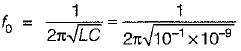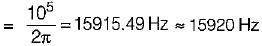QUESTION: 2

### Which of the following Is not true for a series RLC resonant circuit?

Solution: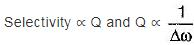When Δω increases, selectivity decreases and vice-versa.
Also, Vc|max occurs at,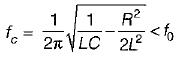QUESTION: 3

### The half-power frequencies of a series resonant circuit where the resonant frequency is 150 x 103 Hz and the bandwidth is 75 kHz will be respectively given by

Solution:

Given, Δf = 75 kHz = f2 - f1...(i)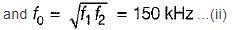On solving equations (i) and (ii), we get
f= 117 kHz and f2 = 192 kHz

QUESTION: 4

For the tank circuit shown below, the circulating current at resonance is given by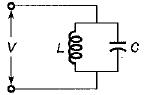Solution:

At resonance, XL = XC
∴ IL = IC (for parallel resonant circuit)
Hence,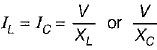or,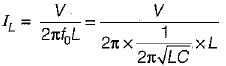or,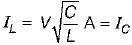(IL=IC = circulating current)

QUESTION: 5

The value of RC in the circuit shown below to yield resonance will be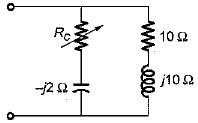Solution:

Here,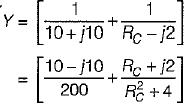At resonance,
Img [Y] = 0
or,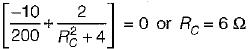QUESTION: 6

A resonating circuit has 10ft resistance, if the supply is 10 Ω, the power at half power frequency will be

Solution:

At resonance,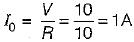Now, power at half power frequency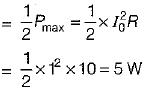QUESTION: 7

For the circuit shown below, what are the values of R1, and R2 so that the circuit will resonate at all frequencies?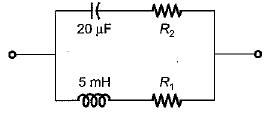Solution:

For the given circuit to resonate at all frequency,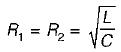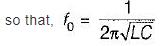Note: Resonant frequency of given circuit is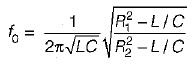Thus,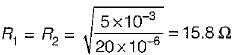QUESTION: 8

The value of current I1 in the circuit shown below is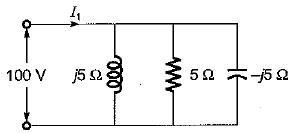Solution:

Since XL = XL, therefore given circuit will be at resonance
∴ I= 100/5 = 20 A

QUESTION: 9

The transfer function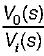of the network shown below is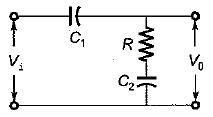Solution:

The given circuit in s-domain is shown below.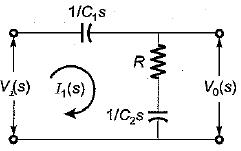Now,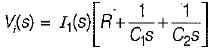and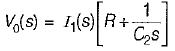So,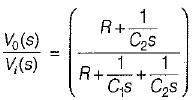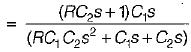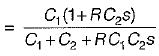or,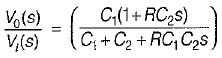QUESTION: 10

The poles and zeros of the transfer function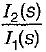for the circuit shown below are located at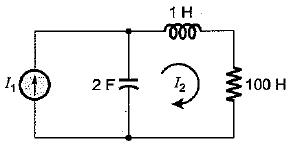Solution: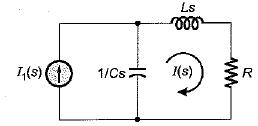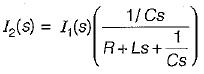or,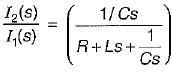or,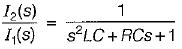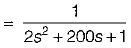Thus, there is no-zero for T.F.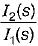Poles are at:
2s2 + 200s + 1 = 0
or,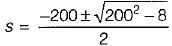or,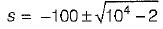= -100 ±99.99
= 0 or -200
Hence, poles are at: s = 0, -200

QUESTION: 11

The output of a linear system for a unit step input is given by t2 e-t. The transfer function is given by

Solution:

Given, C(t) = t2e-t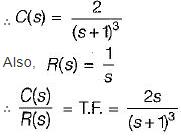QUESTION: 12

A constant voltage but variable frequency ac source feeds L and C in parallel as shown below: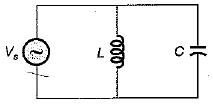The impedance seen by source is Z
1. Z is zero when f = 0.
2. Z is zero when f = infinity.
3. Z is infinite when f = 0.
4. Z is infinite when f = infinity.
5. resonant frequency,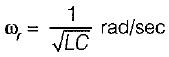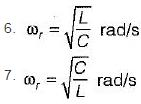From above, the correct answer is

Solution:

Resonant frequency,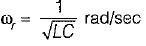Also,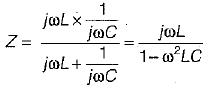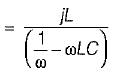When f = 0, ω = 0 and z = 0.
When f = ∞, co = ∞ and z= 0.

QUESTION: 13

Match List- I (Types of filters) with List- II (Attenuation band) and select the correct answer using the codes given below the lists:
List -I
A. Low pass
B. High pass
C. Band pass
D. Band stop

List-II
1. 0 → f2, f1 → ∞
2. fc → ∞
3. f1 → f2
4. 0 → fc

Codes: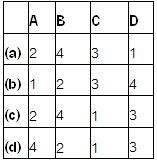Solution:
QUESTION: 14

The transfer function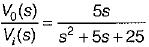is for an active

Solution:

When s → 0 , H (0) → 0
When s → ∞, H(∞) → 0
Hence, given T.F. is for a band pass filter.

QUESTION: 15

Match List- I (Transfer function) with List- II (Type of filter) and select the correct answer using the codes given below the lists: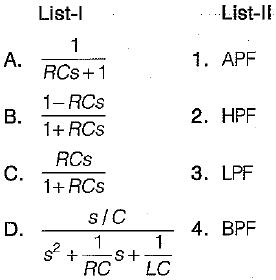Codes: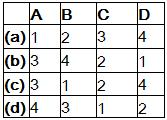Solution:

Putting s → 0 and s → ∞ we can find the type of the filter.